Question

# Given the following reaction: 2CO (?) + O2 (?) → 2CO2 (?) ∆? = −566 kJ/mol...

Given the following reaction:
2CO (?) + O2 (?) → 2CO2 (?) ∆? = −566 kJ/mol
Calculate the change in internal energy when ?∆? at constant pressure is -102 kJ/mol.

By the definitions of thermodynammics, the enthalpy of a system is equal to the sum of its internal energy and the product of its pressure and volume.

i.e.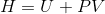Differentiating both sides,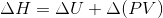As it is mentioned that the system is in constant pressure, so P is a constant value and can be taken out of the differential.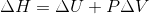Given :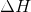= -566 kJ/mol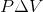= -102 kJ/mol

The negative value ofindicates that the reaction is exothermic, i.e. heat is given out during the reaction.

The negative value ofindicates that the system is getting compressed. This is evident from the fact that 3 moles of gaseous reactants give rise to only 2 moles of gaseous products.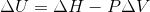= -566 kJ/mol - ( -102 kJ/mol)

= -566 kJ/mol + 102 kJ/mol

= -464 kJ/mol

The negative value of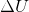indicates that the internal energy of the system has decreased. This is logical as a huge amount of energy has been given out of the system, although the compression had increased the internal energy of the system by a small amount.

#### Earn Coins

Coins can be redeemed for fabulous gifts.

Similar Homework Help Questions
• ### For the reaction 2CO(g)+O2(g)⇌2CO2(g), the value of K at a certain temperature is 1300. Calculate [CO2]...

For the reaction 2CO(g)+O2(g)⇌2CO2(g), the value of K at a certain temperature is 1300. Calculate [CO2] at equilibrium when [CO] = 9.0×10−2 mol/L and [O2] = 0.527 mol/L

• ### Consider the following reaction at equilibrium. 2CO2 (g) 2CO (g) + O2 (g) ΔH° = -514...

Consider the following reaction at equilibrium. 2CO2 (g) 2CO (g) + O2 (g) ΔH° = -514 kJ Le Châtelier's principle predicts that the equilibrium partial pressure of CO (g) can be maximized by carrying out the reaction ________. A.) at low temperature and low pressure B.) at low temperature and high pressure C.) at high temperature and low pressure D.) in the presence of solid carbon E.) at high temperature and high pressure

• ### for the reaction 2CO + O2 = 2CO2 it is found that at a certain temperature...

for the reaction 2CO + O2 = 2CO2 it is found that at a certain temperature the concentration are [CO] = 7.2 x 10^-4 M, [O2] = 8.1 x 10^-3 M, and [CO2] = 2.3 x 10-2 M. write out the equilibrium expression and calculate K for the reaction at this temperature? How would the equilibrium position change if CO2 was added?

• ### 1.     The oxidation of carbon monoxide proceeds as follows:                   2CO(g) + O2(g)    2CO2(g) + 559 kJ Which

1.     The oxidation of carbon monoxide proceeds as follows:                   2CO(g) + O2(g)    2CO2(g) + 559 kJ Which of the following will cause an INCREASEin the equilibrium concentration of CO? a. decreasing the pressure of the system at constant temperature b.   adding more O2to the system c.   removing CO2from the system as it is formed d. increasing the pressure of the system at constant temperature e. adding a catalyst

• ### b) - 227 kJ/mol c) + 251 kJ/mol d) - 251 kJ/mol Given the thermochemical equations...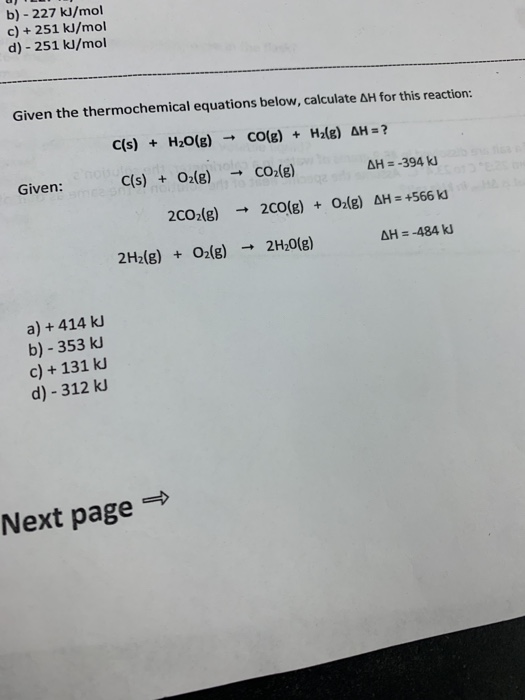b) - 227 kJ/mol c) + 251 kJ/mol d) - 251 kJ/mol Given the thermochemical equations below, calculate AH for this reaction: Given: C(s) + H2O(g) → CO(g) + H2(g) AH = ? C(s) + O2(g) + CO2(g) AH = -394 kJ 2002(g) + 2CO(g) + O2(g) AH = +566 kJ AH = -484 kJ 2H2(g) + O2(g) + 2H20(g) a) + 414 kJ b) - 353 kJ c) + 131 kJ d) - 312 kJ Vext page →

• ### Use the following information and hess's law to find the enthalpy change for the reaction C(g)...

Use the following information and hess's law to find the enthalpy change for the reaction C(g) + O2(g) => CO2(g) reaction 1: 2CO(g) + O2(g) => 2CO2(g) ΔH = -566 kj reaction 2: 2C(g) + O2(g) => 2CO(g) ΔH = -1655 kj

• ### For the following reaction: 2CH4(g) + O2(g) → 2CO(g) + 4H2(g) Compound    ΔH°f (kJ mol-1)...

For the following reaction: 2CH4(g) + O2(g) → 2CO(g) + 4H2(g) Compound    ΔH°f (kJ mol-1)    S° (J mol-1 K-1) CH4 (g)    -74.87    188.66 O2 (g)    0.00    205.70 CO (g)    -110.53    197.66 H2 (g)    0.00    130.68 Calculate ΔG°rx (in kJ) at 345.31 K for this reaction. Report your answer to two decimal places in standard notation (i.e. 123.45 kJ). Assume ΔH°f and S° do not vary as a function of temperature.

• ### Consider the following reaction at equilibrium: 2CO2(g) = 200 (g) + O2(g) AH° = -514 kJ...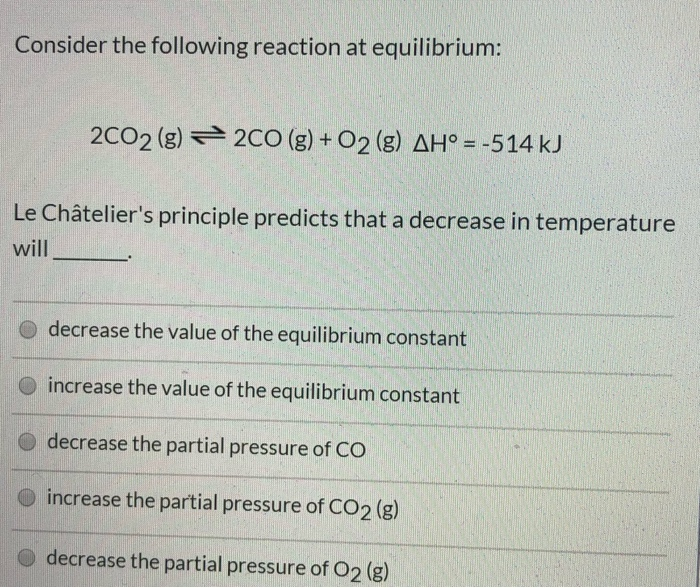Consider the following reaction at equilibrium: 2CO2(g) = 200 (g) + O2(g) AH° = -514 kJ Le Châtelier's principle predicts that a decrease in temperature will decrease the value of the equilibrium constant O increase the value of the equilibrium constant decrease the partial pressure of CO increase the partial pressure of CO2 (g) decrease the partial pressure of O2 (g) Consider the following reaction at equilibrium: 2NH3 (g) = N2 (g) + 3H2(g) AH° = +92.4 kJ Le Châtelier's...

• ### 1. 1) luciferin+O2 ⇌ oxyluciferin+light 2) ATP⇌AMP+PPi ΔG∘=−31.6 kJ/mol If the overall ΔG∘ of the coupled...

1. 1) luciferin+O2 ⇌ oxyluciferin+light 2) ATP⇌AMP+PPi ΔG∘=−31.6 kJ/mol If the overall ΔG∘ of the coupled reaction is -1.21 kJ/mol , what is the equilibrium constant, K, of the first reaction at 11∘C? Round your answer to 3 significant figures. 2. When methanol (CH3OH) is combusted, such as when in a gasoline blend, the following reaction occurs: 2CH3OH(l)+3O2(g)→2CO2(g)+4H2O(g) Based on the standard free energies of formation given, what is the standard free energy change for this reaction? Report the answer...

• ### Using average bond enthalpies (linked above), estimate the enthalpy change for the following reaction: 2CO(g) +...

Using average bond enthalpies (linked above), estimate the enthalpy change for the following reaction: 2CO(g) + 2NO(g)2CO2(g) + N2(g) kJ Bond Bond Energy (kJ/mol) C=C 602 H-H 436 C-H 413 C-C 346 Using average bond enthalpies (linked above), estimate the enthalpy change for the following reaction: I2(g) + Cl2(g)2ICl(g) kJ Using the values of bond energy from the table above, estimate the enthalpy change for the following reaction: C2H4(g) + H2(g) C2H6(g) kJ